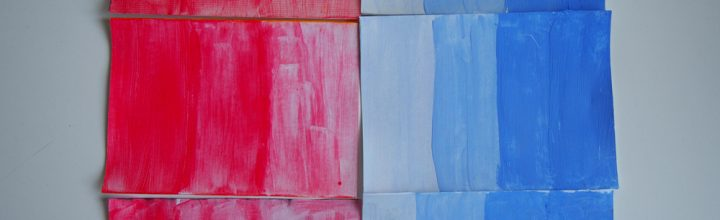# Thermochromic Tests

Posted on Oct 31, 2018 in Circuits, Craft, TutorialsThermochromic pigments change states when you introduce heat. You can control how much it changes by passing current through resistive thread or other resistive materials. Here are a few tests with different threads and resistances.

For more information on how it works, the circuit, and other resources, go here.

Resistance: 10.2 ohms
Code: Full sketch here``` analogWrite(9, 150); delay(2000); analogWrite(9, 0); delay(200); ```

Resistance: 9.1 ohms
Code: Full sketch here``` analogWrite(9, 150); delay(2000); analogWrite(9, 0); delay(200); ```

Resistance: 7.9 ohms
Code: Full sketch here``` analogWrite(9, 150); delay(2000); analogWrite(9, 0); delay(200); ```

Code: Full sketch here``` analogWrite(9, 250); delay(2000); analogWrite(9, 0); delay(400); ```
Code: Full sketch here``` analogWrite(9, 200); delay(2000); analogWrite(9, 0); delay(400); ```
Code: Full sketch here``` analogWrite(9, 200); delay(2000); analogWrite(9, 0); delay(200); ```